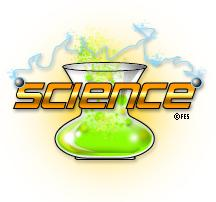## Nemo Vista## High School

Working...### Ms. Wilson

#### SCIENCEClasses Chemistry Physics Physical Science

Calculators are required, they do not need to be graphing calculators but they need to be scientific.

Paper, pencils and a notebook will also be needed.

Physical Science- Heat and temperature will be the topic of discussion. The students will learn what those are and about different temperature scales used in science.

click on the link below to view the frameworks

Chemistry- topic is electrons and their arrangement in the atom

click on the link below to view the frameworks

chemistry frameworks-The performance expectations in Topic 1: Matter and Chemical Reactions help students answer these questions: • How can the structure and properties of matter be explained? • How do substances combine or change (react) to make new substances? • How can patterns be used to characterize and predict chemical reactions? Students develop an understanding of the substructure of atoms and provide more mechanistic explanations of the properties of substances. Students learn how to use the periodic table as a tool to explain and predict the properties of elements. Chemical reactions, including rates of reactions and energy changes, can be understood by students at this level in terms of the collisions of molecules and the rearrangements of atoms. Using this expanded knowledge of chemical reactions, students are able to explain important biological and geophysical phenomena. Students apply an understanding of the process of optimization in engineering design to chemical reaction systems.

Physics- the topic is Forces. When it comes right down to it, all you have to do is sum the forces ..... F=ma

click on the link below to view the frameworks

Physics frameworks-Strand: Motion and Forces Standard 1: Students shall understand one-dimensional motion. MF.1.P.6 Compare and contrast contact force (e.g., friction) and field forces (e.g., gravitational force) MF.1.P.7 Draw free body diagrams of all forces acting upon an object MF.1.P.8 Calculate the applied forces represented in a free body diagram MF.1.P.9 Apply Newton’s first law of motion to show balanced and unbalanced forces MF.1.P.10 Apply Newton’s second law of motion to solve motion problems that involve constant forces: F = ma MF.1.P.11 Apply Newton’s third law of motion to explain action-reaction pairs MF.1.P.12 Calculate frictional forces (i.e., kinetic and static): n k k F F µ = n s s F F µ = MF.1.P.13 Calculate the magnitude of the force of friction: Ff = µFn

Grading policy-The tests count for 100 points each and the student work and labs count for how ever many points are available. For example if there is a 10 question quiz, it would count as 10 points. This normally works out so that the tests count as approximately 50 % of the total grade and class work, homework and quizzes make up the other 50%.

ATTENDANCE IS PARAMOUNT TO SUCCESS

 Contact info e-mail bwilson@nemo.k12.ar.us school phone 501-893-2811